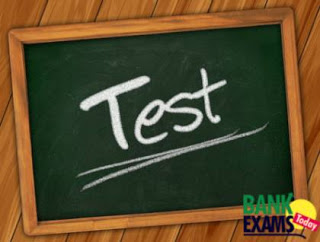# Mixed Aptitude Quiz - 10 (For SBI PO 2016)#### Ques 1.

A librarian purchased 50 story books for his library. But he saw that he could get 14 more books by spending Rs 76 more and average price per book would be reduced by Rs. 1 . The average price (in Rs.) of each book brought was :

Solution :

Total=50+14 = 64
⇒14 = 76+64

14---- 140
1   ----- X

X= 140/14 =10

#### Ques 2.

Seven athletes are participating in a race. In how many ways can the first three prizes be won?

Solution :

⇒  7P3 = 7*6*5=210

#### Ques 3.

A & B together do piece of work in 30 days , B & C together can do it in 20 days. A starts work and works on it for 5 days , then B takes to do the work and works on it for 15 days . Finally C finishes the work in 18 days . The no. of days in which C alone can do the work when doing it separately is

Solution :

Total =60
⇒ A+B=2 Units
⇒ B+C=3 Units
⇒ 5A + 15B + 18 C = 60
⇒ 5(A+B) + 10(B+C)  + 8 C = 60

5*2 + 10*3 + 8C = 60
8c = 20
C = 20/8 = 5/2 = 2.5

⇒ 60/2.5 = 24

#### Ques 4.

In how many ways can the letters of the word ‘GOLDEN’ be arranged?

Solution :

No. of arrangements = 6! = 6×5×4×3×2 = 720

#### Ques 5.

A can do a work in 10 days,B in 15 days. they work for 5 days. rest is done by c in 2 days. if they get 1500 for this work then daily wage of B+c is?

Solution :

5/10 + 5/15 + 2C = 1
⇒ C = 1/12

B + C = 3/20

(3/20) * 1500= 225

#### Ques 6.

A room has length 18 m and breadth 16 m. What will be the total cost if the floor is built at Rs 306 per square metre?

Solution :

Total area of the floor = 18 × 16 = 288 m2

⇒ cost = 288 × 306 = Rs 88128

#### Ques 7.

The speed of a boat in still water is 6 Km/hr and the speed of the stream is 1.5 km/hr . A man rows to a place at a distance of 22.5 km and comes back to the starting point . The total time taken by him is :

Solution :

DownStream speed = 6+1.5 = 7.5 kmph
Upstream Speed = 6-1.5 = 4.5 kmph
Time Take in DownStream =22.5/7.5=3
Time Taken in Upstream = 22.5/4.5=5
Total Time = 3+5=8 hours

#### Ques 8.

man covers a distance on scooter .had he moved 3kmph faster he would have taken 40 min less. If he had moved 2kmph slower he would have taken 40min more.the distance is.

Solution :

3t - 40/60 s = 2
-2t + 40/60 s = 4/3

t = 10/3
S = 12
D = 10/3*12 = 40km

#### Ques 9.

A shopkeeper marked his good 20% more than c.p and give 10% discount on it if he by 100 gm on selling as well as on buying then find profit % ?

Solution :

Sp = 1.2*0.9*1.1 = 118.8
Cp = 90

%profit = (118.8-90) /90 * 100 = 32%

#### Ques 10.

A can do work in 20 and B in 15 days they worked together for 6 days then B replaced by C, A+C does remaining in 4 days. c can do whole work in?

Solution :

A=20
B= 15
LCM = 60
A= 3 Units
B= 4 Units
TOTAL = 7
A+B =  6 ( 20+15/20*15) = 7/10

7--------7/10
1 --------- 10

⇒ C completed remaining work =10*4 = 40 days